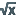You are viewing the RapidMiner Studio documentation for version 9.2 - Check here for latest version

#Create Formula (RapidMiner Studio Core)

## Synopsis

This operator generates a formula from the given model. This operator can generate formula only for models that are capable of producing formula.

## Description

The Create Formula operator extracts a prediction calculation formula from the given model and stores the formula in a formula result object which can then be written into a file, e.g. with the Write operator. Please note that not all RapidMiner models provide a formula and this operator is applicable on only those models that are capable of producing formula.

## Input

•model (Model)

This input port expects a model. The model should be capable of providing a formula.

## Output

•formula (Formula)

The formula of the given model is passed to the output through this port.

•model (Model)

The model that was given as input is passed without changing to the output through this port. This is usually used to reuse the same model in further operators or to view the model in the Results Workspace.

## Tutorial Processes

### Formula of the Logistic Regression model

The 'Ripley-Set' data set is loaded using the Retrieve operator. The Logistic Regression operator is applied on this ExampleSet with default values of all parameters. The regression model generated by the Logistic Regression operator is provided as input to the Create Formula operator which returns a formula object. You can view this formula object in the Results Workspace. It is important to note that most RapidMiner operators do not provide a formula, thus this operator cannot be applied on them.